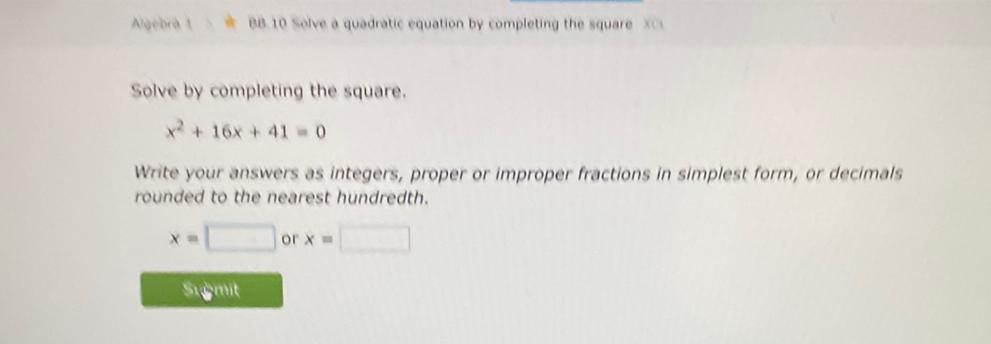Question:

# Solve by completing the square. X^2 + 16x +41 = 0 Write your answers as integers, proper or improper fractions in simplest form, or decimals rounded to the nearest hundredth.Solve by completing the square. X^2 + 16x +41 = 0 Write your answers as integers, proper or improper fractions in simplest form, or decimals rounded to the nearest hundredth.